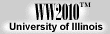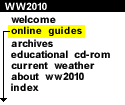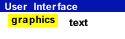.
Pressure Activity
teacher guide

1) The units are inches of Mercury ("Hg), atmospheres (atm), kilopascals (kPa) and millibars (mb). Millibars is the unit used most by meteorologists.

2) Decreases because the number of molecules above a surface decreases with height. Since there are fewer air molecules at higher levels to exert a force on a surface, the pressure at higher levels is less than at lower levels.

3) A blue "H" represents a high pressure center. A red "L" represents a low pressure center.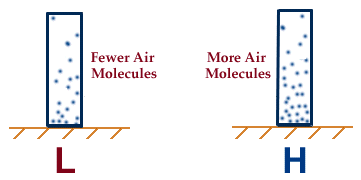4) A low pressure center should be labeled beneath the left column because there are fewer air molecules in that column to exert a force on the surface below. Therefore, the pressure at the surface is lower (than for the right column).

5) The green contours are isobars and they are lines of constant pressure.6) Working with current weather data, so no answer key is available.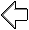introduction
 Terms for using data resources. CD-ROM available. Credits and Acknowledgments for WW2010. Department of Atmospheric Sciences (DAS) atthe University of Illinois at Urbana-Champaign.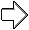air masses